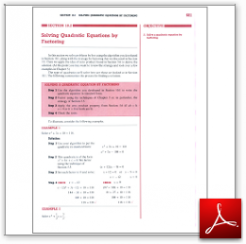Algebra Math Book - Introductory Algebra Thank you for your continual support.

 The power of mathematics rests in the logic of thinking.ID: Sec10-2
Price: 1.99

# Algebra Math book - Introductory Algebra - Chapter 10 - Section 2 - Solving Quadratic Equations by Factoring

## Section 10.2 - Objectives

2.  Solve a quadratic equation by factoring.

Navigate to
Previous Section or Next Section
or Chapter 10 Details or Other Chapters

This section of my algebra math book, Introductory Algebra, also includes in the download:

• Cover Sheet